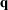International Tables for Crystallography (2006). Vol. D, ch. 2.3, pp. 314-328https://doi.org/10.1107/97809553602060000640

## Contents

• 2.3. Raman scattering  (pp. 314-328)
• 2.3.1. Introduction  (p. 314) | html | pdf |
• 2.3.2. Inelastic light scattering in crystals – basic notions  (pp. 314-315) | html | pdf |
• 2.3.2.1. Kinematics  (p. 314) | html | pdf |
• 2.3.2.2. Cross section  (pp. 314-315) | html | pdf |
• 2.3.2.3. Experimental aspects  (p. 315) | html | pdf |
• 2.3.3. First-order scattering by phonons  (pp. 315-322) | html | pdf |
• 2.3.3.1. First-order scattering cross section and Raman spectral line shapes  (p. 316) | html | pdf |
• 2.3.3.2. Symmetry properties of the scattering cross section  (pp. 316-317) | html | pdf |
• 2.3.3.3. Raman tensor and selection rules at(pp. 317-319) | html | pdf |
• 2.3.3.4. Centrosymmetric crystals  (pp. 319-321) | html | pdf |
• 2.3.3.5. Noncentrosymmetric crystals  (pp. 321-322) | html | pdf |
• 2.3.3.6.-dependent terms  (p. 322) | html | pdf |
• 2.3.4. Morphic effects in Raman scattering  (pp. 322-325) | html | pdf |
• 2.3.4.1. General remarks  (pp. 322-323) | html | pdf |
• 2.3.4.2. Electric-field-induced scattering  (pp. 323-324) | html | pdf |
• 2.3.4.3. Raman scattering in a magnetic field  (pp. 324-325) | html | pdf |
• 2.3.4.4. Stress- (strain-) induced Raman scattering  (p. 325) | html | pdf |
• 2.3.5. Spatial-dispersion effects  (pp. 325-326) | html | pdf |
• 2.3.6. Higher-order scattering  (pp. 326-327) | html | pdf |
• 2.3.7. Conclusions  (pp. 327-328) | html | pdf |
• 2.3.8. Glossary  (p. 328) | html | pdf |
• References | html | pdf |
• Tables
• Table 2.3.3.1. Symmetry of Raman tensors in the 32 crystal classes  (pp. 318-320) | html | pdf |
• Table 2.3.3.2. Raman selection rules in crystals of theclass  (p. 321) | html | pdf |
• Table 2.3.3.3. Raman selection rules in crystals of the 4 mm class  (p. 322) | html | pdf |
• Table 2.3.4.1. Symmetrized ( s ) and antisymmetrized ( a ) sets of trilinear basis functions corresponding to symmetry species of the 4 mm class  (p. 323) | html | pdf |
• Table 2.3.6.1. Thermal factors for second-order Raman scattering  (p. 327) | html | pdf |